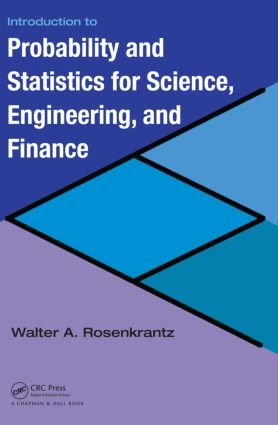# Introduction to Probability and Statistics for Science, Engineering, and Finance

## 1st Edition

Chapman and Hall/CRC

680 pages | 99 B/W Illus.

Hardback: 9781584888123
pub: 2008-07-10
SAVE ~\$24.00
\$120.00
\$96.00
x
eBook (VitalSource) : 9780429142048
pub: 2008-07-10
from \$60.00

FREE Standard Shipping!

### Description

Integrating interesting and widely used concepts of financial engineering into traditional statistics courses, Introduction to Probability and Statistics for Science, Engineering, and Finance illustrates the role and scope of statistics and probability in various fields.

The text first introduces the basics needed to understand and create tables and graphs produced by standard statistical software packages, such as Minitab, SAS, and JMP. It then takes students through the traditional topics of a first course in statistics. Novel features include:

• Applications of standard statistical concepts and methods to the analysis and interpretation of financial data, such as risks and returns
• Cox–Ross–Rubinstein (CRR) model, also called the binomial lattice model, of stock price fluctuations
• An application of the central limit theorem to the CRR model that yields the lognormal distribution for stock prices and the famous Black–Scholes option pricing formula
• An introduction to modern portfolio theory
• Mean-standard deviation diagram of a collection of portfolios
• Computing a stock’s betavia simple linear regression
• As soon as he develops the statistical concepts, the author presents applications to engineering, such as queuing theory, reliability theory, and acceptance sampling; computer science; public health; and finance. Using both statistical software packages and scientific calculators, he reinforces fundamental concepts with numerous examples.

### Reviews

"The book provides a very well-written, comprehensive treatment of all the standard requirements for an introductory course … Summing Up: Highly recommended."

CHOICE, February 2009

Data Analysis

Orientation

The Role and Scope of Statistics in Science and Engineering

Types of Data: Examples from Engineering, Public Health, and Finance

The Frequency Distribution of a Variable Defined on a Population

Quantiles of a Distribution

Measures of Location (Central Value) and Variability

Covariance, Correlation, and Regression: Computing a Stock’s Beta

Mathematical Details and Derivations

Large Data Sets

Probability Theory

Orientation

Sample Space, Events, Axioms of Probability Theory

Mathematical Models of Random Sampling

Conditional Probability and Bayes’ Theorem

The Binomial Theorem

Discrete Random Variables and Their Distribution Functions

Orientation

Discrete Random Variables

Expected Value and Variance of a Random Variable

The Hypergeometric Distribution

The Binomial Distribution

The Poisson Distribution

Moment Generating Function: Discrete Random Variables

Mathematical Details and Derivations

Continuous Random Variables and Their Distribution Functions

Orientation

Random Variables with Continuous Distribution Functions: Definition and Examples

Expected Value, Moments, and Variance of a Continuous Random Variable

Moment Generating Function: Continuous Random Variables

The Normal Distribution: Definition and Basic Properties

The Lognormal Distribution: A Model for the Distribution of Stock Prices

The Normal Approximation to the Binomial Distribution

Other Important Continuous Distributions

Functions of a Random Variable

Mathematical Details and Derivations

Multivariate Probability Distributions

Orientation

The Joint Distribution Function: Discrete Random Variables

The Multinomial Distribution

Mean and Variance of a Sum of Random Variables

Why Stock Prices Have a Lognormal Distribution: An Application of the Central Limit Theorem

Modern Portfolio Theory

Risk Free and Risky Investing

Theory of Single and Multi-Period Binomial Options

Black–Scholes Formula for Multi-Period Binomial Options

The Poisson Process

Applications of Bernoulli Random Variables to Reliability Theory

The Joint Distribution Function: Continuous Random Variables

Mathematical Details and Derivations

Sampling Distribution Theory

Orientation

Sampling from a Normal Distribution

The Distribution of the Sample Variance

Mathematical Details and Derivations

Point and Interval Estimation

Orientation

Estimating Population Parameters: Methods and Examples

Confidence Intervals for the Mean and Variance

Point and Interval Estimation for the Difference of Two Means

Point and Interval Estimation for a Population Proportion

Some Methods of Estimation

Hypothesis Testing

Orientation

Tests of Statistical Hypotheses: Basic Concepts and Examples

Comparing Two Populations

Normal Probability Plots

Tests Concerning the Parameter p of a Binomial Distribution

Statistical Analysis of Categorical Data

Orientation

Chi Square Tests

Contingency Tables

Linear Regression and Correlation

Orientation

Method of Least Squares

The Simple Linear Regression Model

Model Checking

Correlation Analysis

Mathematical Details and Derivations

Large Data Sets

Multiple Linear Regression

Orientation

The Matrix Approach to Simple Linear Regression

The Matrix Approach to Multiple Linear Regression

Mathematical Details and Derivations

Single-Factor Experiments: Analysis of Variance

Orientation

The Single Factor ANOVA Model

Confidence Intervals for the Treatment Means; Contrasts

Random Effects Model

Mathematical Derivations and Details

Design and Analysis of Multi-Factor Experiments

Orientation

Randomized Complete Block Designs

Two-Factor Experiments with n > 1 Observations per Cell

2k Factorial Designs

Statistical Quality Control

Orientation

x and R Control Charts

p charts and c charts

Appendix: Tables

Index

Chapter Summary, Problems, and To Probe Further sections appear at the end of each chapter.